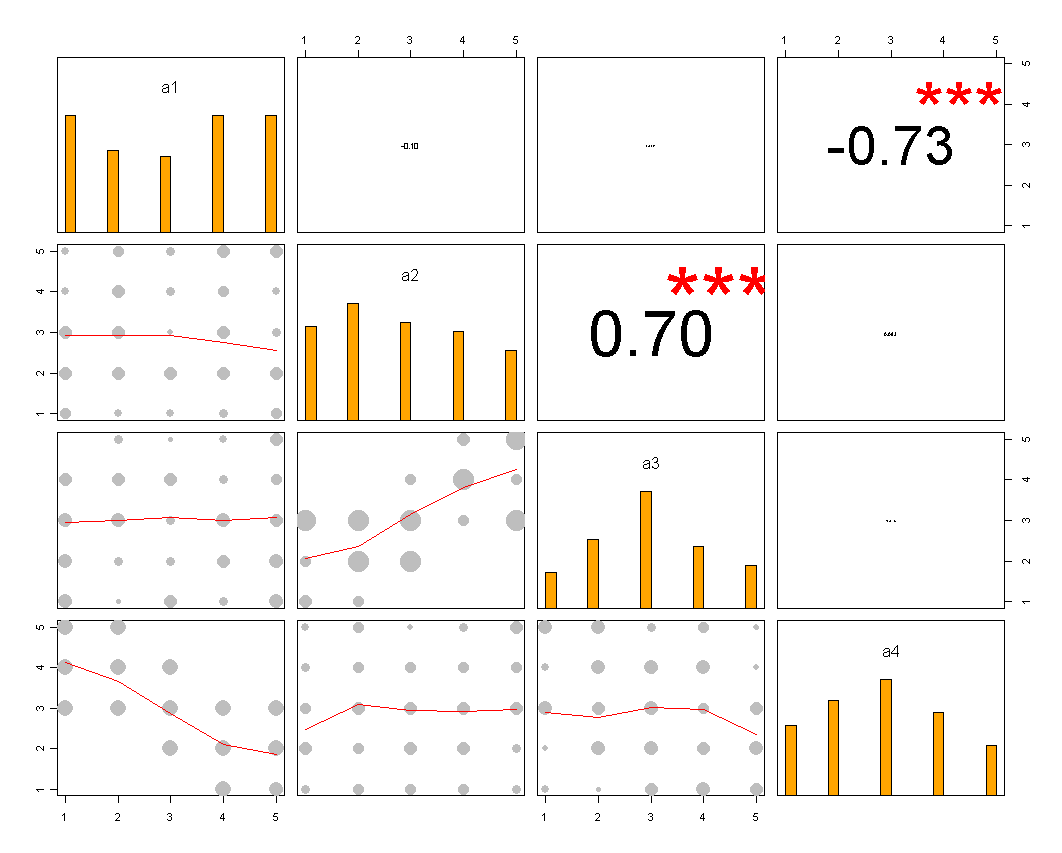Want to share your content on R-bloggers? click here if you have a blog, or here if you don't.

When analyzing a questionnaire, one often wants to view the correlation between two or more Likert questionnaire item’s (for example: two ordered categorical vectors ranging from 1 to 5).

When dealing with several such Likert variable’s, a clear presentation of all the pairwise relation’s between our variable can be achieved by inspecting the (Spearman) correlation matrix (easily achieved in R by using the “cor.test” command on a matrix of variables).
Yet, a challenge appears once we wish to plot this correlation matrix. The challenge stems from the fact that the classic presentation for a correlation matrix is a scatter plot matrix – but scatter plots don’t (usually) work well for ordered categorical vectors since the dots on the scatter plot often overlap each other.

There are four solution for the point-overlap problem that I know of:

1. Jitter the data a bit to give a sense of the “density” of the points
2. Use a color spectrum to represent when a point actually represent “many points”
3. Use different points sizes to represent when there are “many points” in the location of that point
4. Add a LOWESS (or LOESS) line to the scatter plot – to show the trend of the data

In this post I will offer the code for the  a solution that uses solution 3-4 (and possibly 2, please read this post comments). Here is the output (click to see a larger image):And here is the code to produce this plot:

### R code for producing a Correlation scatter-plot matrix – for ordered-categorical data

Note that this code will work fine for continues data points (although I might suggest to enlarge the “point.size.rescale” parameter to something bigger then 1.5 in the “panel.smooth.ordered.categorical” function)

```1 2 3 4 5 6 7 8 9 10 11 12 13 14 15 16 17 18 19 20 21 22 23 24 25 26 27 28 29 30 31 32 33 34 35 36 37 38 39 40 41 42 43 44 45 46 47 48 49 50 51 52 53 54 55 56 57 58 59 60 61 62 63 64 65 66 67 68 69 70 71 72 73 74 75 76 77 78 79 80 81 82 83 84 85 86 87 88 89 90 91 92 93 94 # ----------------- # Functions # -----------------   panel.cor.ordered.categorical <- function(x, y, digits=2, prefix="", cex.cor) {   usr <- par("usr"); on.exit(par(usr)) par(usr = c(0, 1, 0, 1))   r <- abs(cor(x, y, method = "spearman")) # notive we use spearman, non parametric correlation here r.no.abs <- cor(x, y, method = "spearman")     txt <- format(c(r.no.abs , 0.123456789), digits=digits) txt <- paste(prefix, txt, sep="") if(missing(cex.cor)) cex <- 0.8/strwidth(txt)   test <- cor.test(x,y, method = "spearman") # borrowed from printCoefmat Signif <- symnum(test\$p.value, corr = FALSE, na = FALSE, cutpoints = c(0, 0.001, 0.01, 0.05, 0.1, 1), symbols = c("***", "**", "*", ".", " "))   text(0.5, 0.5, txt, cex = cex * r) text(.8, .8, Signif, cex=cex, col=2) }         panel.smooth.ordered.categorical <- function (x, y, col = par("col"), bg = NA, pch = par("pch"), cex = 1, col.smooth = "red", span = 2/3, iter = 3, point.size.rescale = 1.5, ...) { #require(colorspace) require(reshape) z <- merge(data.frame(x,y), melt(table(x ,y)),sort =F)\$value #the.col <- heat_hcl(length(x))[z] z <- point.size.rescale*z/ (length(x)) # notice how we rescale the dots accourding to the maximum z could have gotten   symbols( x, y, circles = z,#rep(0.1, length(x)), #sample(1:2, length(x), replace = T) , inches=F, bg= "grey",#the.col , fg = bg, add = T)   # points(x, y, pch = pch, col = col, bg = bg, cex = cex) ok <- is.finite(x) & is.finite(y) if (any(ok)) lines(stats::lowess(x[ok], y[ok], f = span, iter = iter), col = col.smooth, ...) }     panel.hist <- function(x, ...) { usr <- par("usr"); on.exit(par(usr)) par(usr = c(usr[1:2], 0, 1.5) ) h <- hist(x, plot = FALSE, br = 20) breaks <- h\$breaks; nB <- length(breaks) y <- h\$counts; y <- y/max(y) rect(breaks[-nB], 0, breaks[-1], y, col="orange", ...) }     pairs.ordered.categorical <- function(xx,...) { pairs(xx , diag.panel = panel.hist , lower.panel=panel.smooth.ordered.categorical, upper.panel=panel.cor.ordered.categorical, cex.labels = 1.5, ...) }         # ----------------- # Example # -----------------   set.seed(666) a1 <- sample(1:5, 100, replace = T) a2 <- sample(1:5, 100, replace = T) a3 <- round(jitter(a2, 7) ) a3[a3 < 1 | a3 > 5] <- 3 a4 <- 6-round(jitter(a1, 7) ) a4[a4 < 1 | a4 > 5] <- 3   aa <- data.frame(a1,a2,a3, a4)   require(reshape)   # plotting :) pairs.ordered.categorical(aa)```

### Credits:

• The original R code for the correlation matrix plot was taken from R Graph Gallery (The differences are: 1) The use of spearman correlation; 2) The adding of hist panel and; 3) The changing of points sizes
• The idea to use symbols for changing the point sizes was offered by Doug Y’barbo.
And also to Dirk Eddelbuettel for offering to use cex (although I ended up not using that)

If you got ideas on how to improve this code (or reproducing it with ggplot2 or lattice), please do so in the comments (or on your own blog, but be sure to let me know)